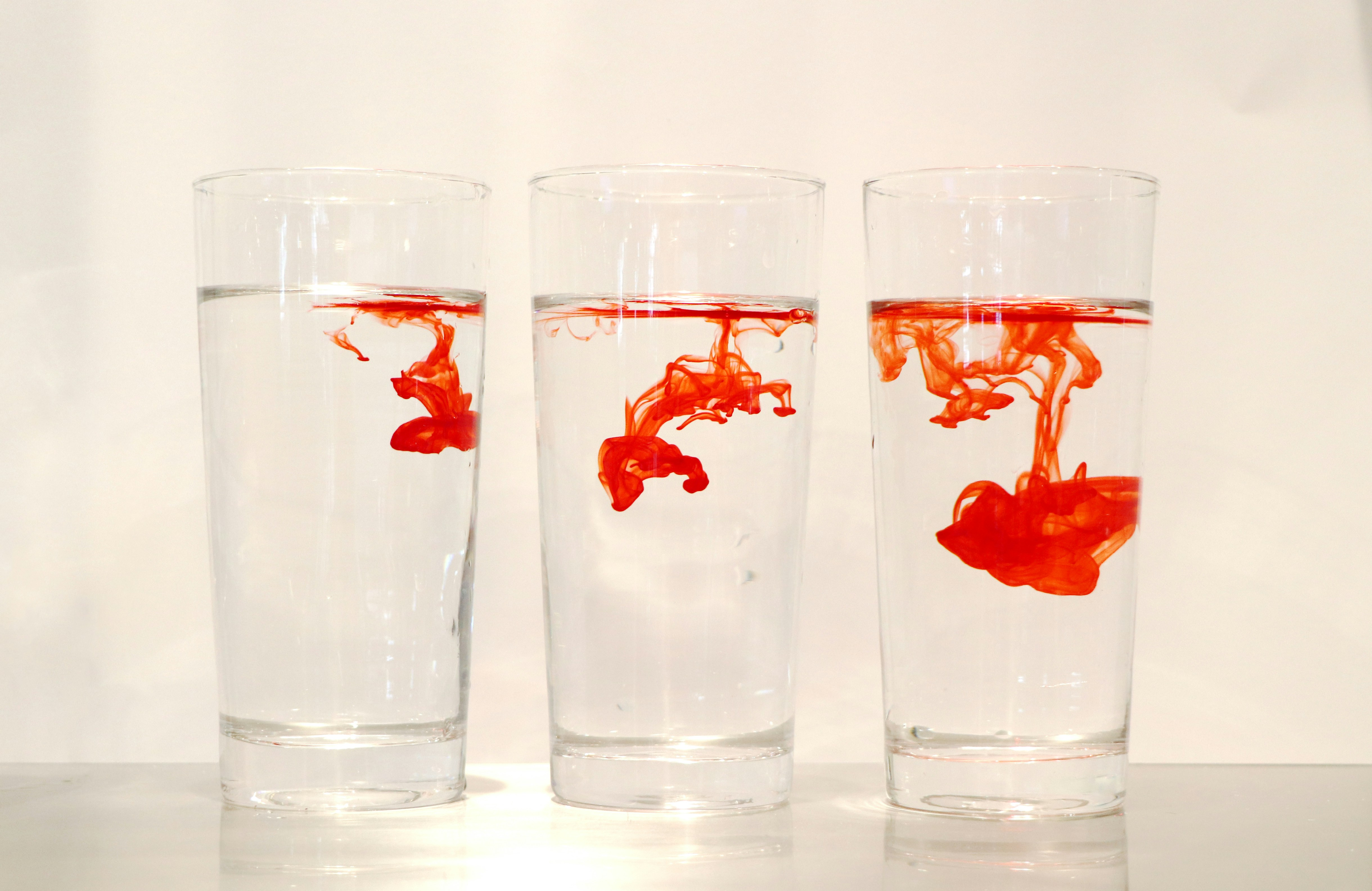September 27, 2020

# DiffWave and WaveGrad: Overview (Part 1)

DiffWave and WaveGrad propose a new neural vocoder model based on diffusion probabilistic processes, with several nice properties and a solid theoretical justification.This is the first part of a two part blog post. If you've read this, move on to Part 2!

Two recent papers, DiffWave (NVidia) and WaveGrad (Google), propose a new neural vocoder model based on diffusion probabilistic processes. These vocoders have several nice properties – they achieve high quality synthesis, are non-autoregressive, are easy to train (no adversarial losses), do extremely well on unconditional audio synthesis, and are likely fast enough for eventual production deployment on GPUs.

These models are conceptually simple, but come with a fairly hard-to-parse theoretical justification. In this blog post, I'd like to go over how these models work at a high level, and then dive in to the theoretical justification for them.

### Training

The model itself is a neural denoising autoencoder.

To train it, start with a clean audio signal $x$ and a sample of white noise $\epsilon \sim N(0, I)$. Then, create a corrupted audio signal $\tilde x$ by scaling the noise to have variance $\sigma^2$ and adding it to the clean audio:

$$\tilde x = \sqrt{1 - \sigma^2} x + \sigma \epsilon$$

If we scale $x$ by $\sqrt{1 - \sigma^2}$, the variance of $\tilde x$ is unchanged from the original audio, if your input is unit variance.

Then, train a neural network $f_\theta$ to predict the noise that was added with an L2 loss:

$$J(\theta) = \left(f_\theta(\tilde x, \sigma) - \epsilon\right)^2$$

The network $f_\theta$ is conditioned on the noise magnitude $\sigma$. (WaveGrad uses an L1 loss here, finding that it "offers better training stability".) This conditioning is important, as we will use different values of $\sigma$ throughout training. In a conditional synthesis setting, $f_\theta$ is also conditioned upon any input features such as linguistic information or mel spectrograms (but I won't write that explicitly here).

### Inference

To use this model for inference, we will perform $T$ steps of denoising with sampling. First, we sample a starting point $y_T$ of white noise. Then, each step of denoising is computed with:

$$y_{t-1} = c_1 y_t - c_2 f_\theta(y_t) + \sigma_t z,$$

where $z \sim N(0, I)$. In essence, each step reduces the magnitude of the signal (scaling by $c_1$), removes some noise (subtracting $f_\theta(y_t)$), and samples some noise to add to the signal of variance $\sigma^2$ (with gradually decreasing $\sigma^2$ and $\sigma_1 = 0$).

The result, $y_0$, is the final audio.

Choosing values for $c_1$, $c_2$, and $\sigma_t$ depends on the theoretical justification for this model. In the WaveGrad paper, Section 2 describes an interpretation based on sampling via Langevin dynamics, in which case $c_1 = 1$, $c_2 = \frac{{\sigma_t}^2}{2}$, and $\sigma_t$ is any sequence of magnitudes that is sufficiently long and gradually decreasing.

However, both WaveGrad and DiffWave choose these values based instead on the interpretation of diffusion probabilistic processes, and according to one of the authors of DiffWave, the exact values are important for high-quality synthesis.

In Part 2, I'll dig into the somewhat gnarly math required to justify all of this, ignoring the Langevin dynamics interpretation entirely.